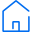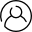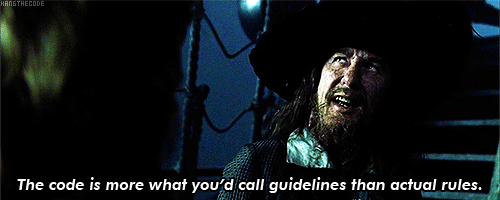# EvA

Eva is a Java OOP implementation of an evolutionary algorithm, which is a concept from the field of artifical intelligence. It can help find solutions to complicated problems that otherwise would require very much computational effort to be solved.

### Examples of problems that can be solved:

• The backpack problem [wiki]
• The salesman's problem [wiki]
• ... and really any other problem for which you can
formalize the solution, solution generation and solution evaluation,
in such a way that Eva can understand (see Usage page).

### How does it work?

First, a number of possible solutions are generated and evaluated (evaluating a solution means saying how good, or how "fit" it is). Based on these solutions, with each iteration, it tries to create better solutions by changing and combining the existing ones until finally, a solution is chosen to be the answer. The algorithm runs until certain conditions are met, like:

• number of iterations (implicit)
• time consumed
• a solution with a minimal "fitness" was found etc.

### Wait, what?

From the simplicity of the above paragraph, you might have guessed that Eva is a heuristic, rather than an algorithm. It's called an agorithm in AI, because it tries to follow the evolving steps of living organisms (if you dig deeper in the theory, you will find words like "chromosome", or "mutation").In other words, run it with the same input multiple times and you most likely won't get the same output. Also, based on the conditions you set, you might get a very satisfying solution, or one that is very bad.

Having this in sight, you must know not to use this method where both precision and speed are required. Depending on your problem, you might guarantee a satisfying result by specifying appropriate stopping conditions, but that takes away the speed. For a big input and strict conditions, it can take a lot of time for the algorithm to end - this is influenced also by the machine's specifications, of course.